Ricci-flat Invariant Geometry in Four Dimensions

On 4-dimensional Lie-Groups that bear a left-invariant metric so that the Ricci-curvature tensor vanishes but the Riemannian curvature is non-zero.

Introduction

In the article Invariants of real low dimensional Lie-algebras, the authors J. Patera, R. Sharp, P. Winternitz, and H. Zassenhaus state a classification of 4-dimensional Lie-algebras. The classification comprises of 12 types of pairwise non-isomorphic algebras. Several of those types depend on one or more real parameters.

A left-invariant metric on a Lie-group is determined by a single scalar product defined on the tangent space of a single point of the Lie-group. We define the scalar product on the tangent space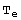G with respect to the same basis used to define the Lie-algebra onG. The coefficients we choose for the scalar product are arranged as

B=(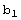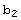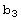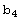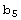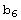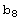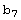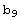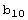)

We aim to find all pairs (g,B), where g is a 4-dimensional Lie-algebra that corresponds to a Lie-group with left-invariant metric g, and in the neutral element e∈G the metric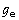=B coincides with the scalar product B.

Our strategy is to investigate all 12 types of Lie-algebras in the classification of J. Patera, R. Sharp, and P.Winternitz reproduced in their paper Invariants of real low dimension Lie algebras. For each such Lie-algebra we initially assume a general scalar product B onG. Then, the entries of the Ricci- and Riemannian-curvature tensors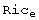and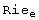onG are polynomials in the coefficients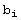of the scalar product B. With respect to the left-invariant metric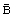on G, we demand the following algebraic equations

 det B≠0,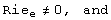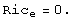We employ Mathematica to solve the equations, i.e. to obtain restrictions on the coefficients. However, depending on the Lie-algebra structure, the equations are quite difficult to solve. Among the 12 types of Lie-algebras there remain 4 types that we are able to solve at all.

A useful reference is the paper Four-dimensional Pseudo-Riemannian Homogeneous Spaces by B. Komrakov. The author explains how=B relates to. Alternatively, have a look at Section 1.4 of my Thesis On Lorentzian Ricci-flat homogeneous manifolds by Jan P. Hakenberg.

Investigations of pairs

We investigate individually all 12 types of Lie-algebras {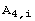:i=1,2,...,12} in the classification of J. Patera, R. Sharp, and P.Winternitz reproduced in their paper Invariants of real low dimension Lie algebras. For each such Lie-algebra g=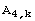we initially assume a general scalar product B onG. All geometric considerations are local, and with respect to the left-invariant metricon G.

At this point the scalar product B has 10 coefficients. Whenever possible, we simplify B by applying suitable automorphisms of the Lie-algebra g to B. An automorphisms grants the isomorphy (isometry) of two pairs (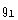,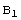) and (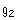,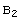). In some of the twelve cases this reduction helps considerably from the computational point of view.

Technically, we ask Mathematica to grant Ricci-flatness and a non-degenerated scalar-product,=0, and det B≠0. The homogeneous pairs (g,B) that are necessarily Riemannian-flat are dropped in the next chapter.

Summary of ricci-flat pairs

In the previous section, for each pair {(,B):i=1,2,...,12} we have imposed=0, and naturally det B≠0. Below, we summarize all pairs (g,B) featuring this geometry that we have collected over the course of investigation.

Specifically, our results are exhaustive for the cases i∈I:={1,2,3,4,7,8,10,12}. That means, any Ricci-flat non-Riemannian-flat pair {(,B):i∈I} is isomorphic to one pair listed below.

Isomorphy types of ricci-flat pairs

This final section demonstrates how to further classify the ricci-flat pairs for the case (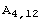,B). The other pairs are much more complicated.

Future work

One investigates the remaining pairs (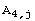,B) with j∈J:={5,6,9,11} exhaustively.

One classifies the ricci-flat pairs up to isomorphy to simplify the visual representation.

One distinguishes the signatures of the scalar-products. For instance, any Ricci-flat non-Riemannian-flat pairs of type (,B) has even signature, because |B|>0. This observation gives rise to the conjecture, that all existing pairs are already covered by my diploma thesis.

I hope to collaborate with Thomas Neukirchner on these issues.

 Created by Mathematica  (September 15, 2007)